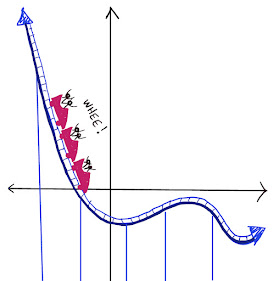## Wednesday, November 30, 2022

### Why can't I divide by zero?

What is 4 divided by zero?  Some students reason that you can think of it as long division and take out as many zeroes as you like from 4 without ever making 4 smaller.  So maybe 4 divided by zero is "infinity"?

That's not unreasonable.  In fact American textbooks from the 1800s took that point of view.  The image below is from the 1876 edition of Charles Davies algebra textbook, p. 83. He says that a number divided by zero is equivalent to infinity.

The problem with dividing by zero is that it leads to contradictions. If 4 divided by zero is "infinity," then does that mean that infinity times zero equals 4?

Check out this sweet little song about Zero, which shows the problem.  Zero is powerful, but it is also dangerous. If you are not careful when you divide, you might run into contradictions. You might even break math! Link to video is here.

## Wednesday, November 9, 2022

### Rectangles and Triangles

Rectangles are so simple.  Their angles are always 90 degrees.  But triangles come in all different shapes. So how do you find the area of a triangle?

Check out this musical video about the area of triangles:  Rectangles and Triangles

### PLAYFUL MATH BLOG CARNIVAL #163

BLOG CARNIVAL #163....LET'S GO! Fun fact: The number 163 is prime, which we can prove simply by showing that it is not divisible by 2, 3...